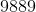ISEE Middle Level Math : Data Analysis

Example Questions

Example Question #121 : Data Analysis And Probability

Use the following table to answer the question: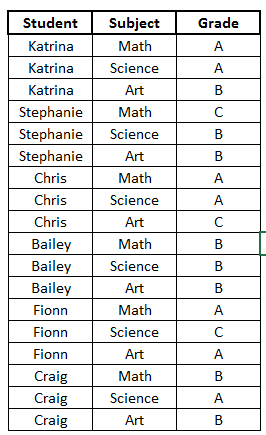What grade did Chris receive in Science?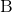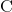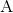Explanation:

To answer the question, let's look at the table.We can see the first column shows the different students, the next column shows the classes they are in, and the last column shows their grade in the class.

Now, to find what grade Chris received in Science, we will first locate Chris' name in the first column.

Now, we can see his name appears 3 times.  If we look to the right, we can see his name matches up to 3 different classes:  Math, Science, and Art.  We want to know his grade in Science, so we will choose that row.

We will again look to the right to see the grade.  We can see that it says A.

Therefore, the grade that Chris received in Science was an A.

Example Question #13 : Tables

Below is the list of candidates for Student Council president, along with the number of votes each won: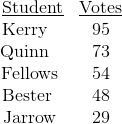What percent of the students voted for Jarrow (nearest tenth)?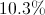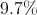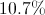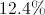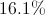Explanation: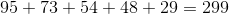students total voted. Out of those, 29 voted for Jarrow. To convert this to a percent, use this proportion and solve for: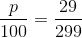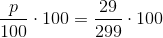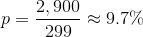Example Question #1 : Mode

On the last day of school,Adam asked each of his classmates how many days of school they missed for the entire school year.  He created a stem-and-leaf plot with the information.

0 | 0 0 2 4 5 6 8

1 | 0 0 1 2

2 | 3

4 | 7

What is the mode(s) of the stem-and-leaf plot?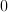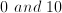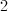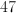Explanation:

The mode of a set of data is the value that occurs the most frequently.

Here, 0 occurs twice on the first line, and twice on the second line.  These are different values since the left side of the vertical line represents the ten's place.  So on the first line, the "0" represents 0, and on the second line the "0" represents 10.  So 0 occurs twice, and ten occurs twice.

It is possible to have more than one mode.  If multiple values occur more than once, occur the same number of times as each other, and occur more than any other values, then they are both modes.

Example Question #2 : Mode

What is the mode of 43, 23, 56, 44, 47, and 43?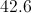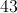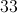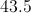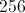Explanation:

The mode is the number that appears most frequently.  All the numbers appear once, except for 43, which occurs twice.  43 is the mode of this number set.

Example Question #3 : Mode

Find the mode: 45, 38, 29, 12, 38, 20, 13.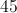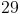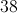Explanation:

Identlfy the number that appears most frequently in the set.

The answer is 38.

Example Question #1 : How To Find Mode

Find the mode: 213, 144, 213, 788, 532, 532, 965, 690, 532, 714.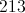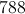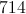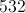Explanation:

Identlfy the number that appears most frequently in the set.

The answer is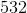.

Example Question #2 : How To Find Mode

Find the mode in this set of numbers:

7435, 8976, 2784, 7435, 1289, 8976, 2110, 8992, 8976, 2784.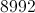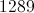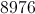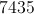Explanation:

Find the most recurring number in the set.

The answer is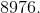Example Question #1 : Mode

Find the mode in this set of numbers:

22, 24, 28, 23, 27, 24, 29, 35, 23, 42, 42, 24, 26, 25, 26.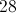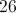Explanation:

Find the number that appears most frequently.

Answer: The mode is 24.

Example Question #1 : How To Find Mode

Fidn the mode in this set of numbers:

112, 121, 123, 132, 121, 211, 120, 122, 121, 131, 133, 113, 311, 121, 211.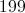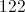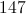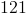Explanation:
 Find the number that appears most frequently.

Answer: The mode is 121.

Example Question #433 : Isee Middle Level (Grades 7 8) Mathematics Achievement

Find the mode in this set of numbers:

9881, 9889, 9818, 9981, 9891, 9918, 9989, 9889, 9198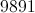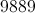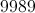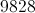Identify the most recurring number in the set: